Next: Theorems Up: Generalized a,b-Fibonacci numbers (this Previous: Generalized a,b-Fibonacci numbers (this   Contents

Definitions

• The generalized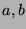-Fibonacci sequence (the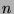th term of which is denoted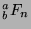) is defined recursively by the following statements:
•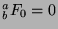.
•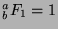.
•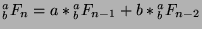,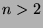.
Note that the regular Fibonacci numbers can be denoted byin this notaion.
•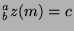means that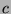has the following properties:
•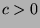.
•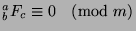.
•is the least positive integer satisfying these properties.
•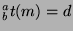means that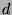has the following properties:
•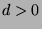.
•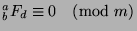.
•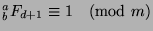.
•is the least positive integer satisfying these properties.
Note: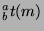is then the function that gives the period of the generalized-Fibonacci sequence in modulo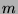.

Gregory Stoll 2000-04-08